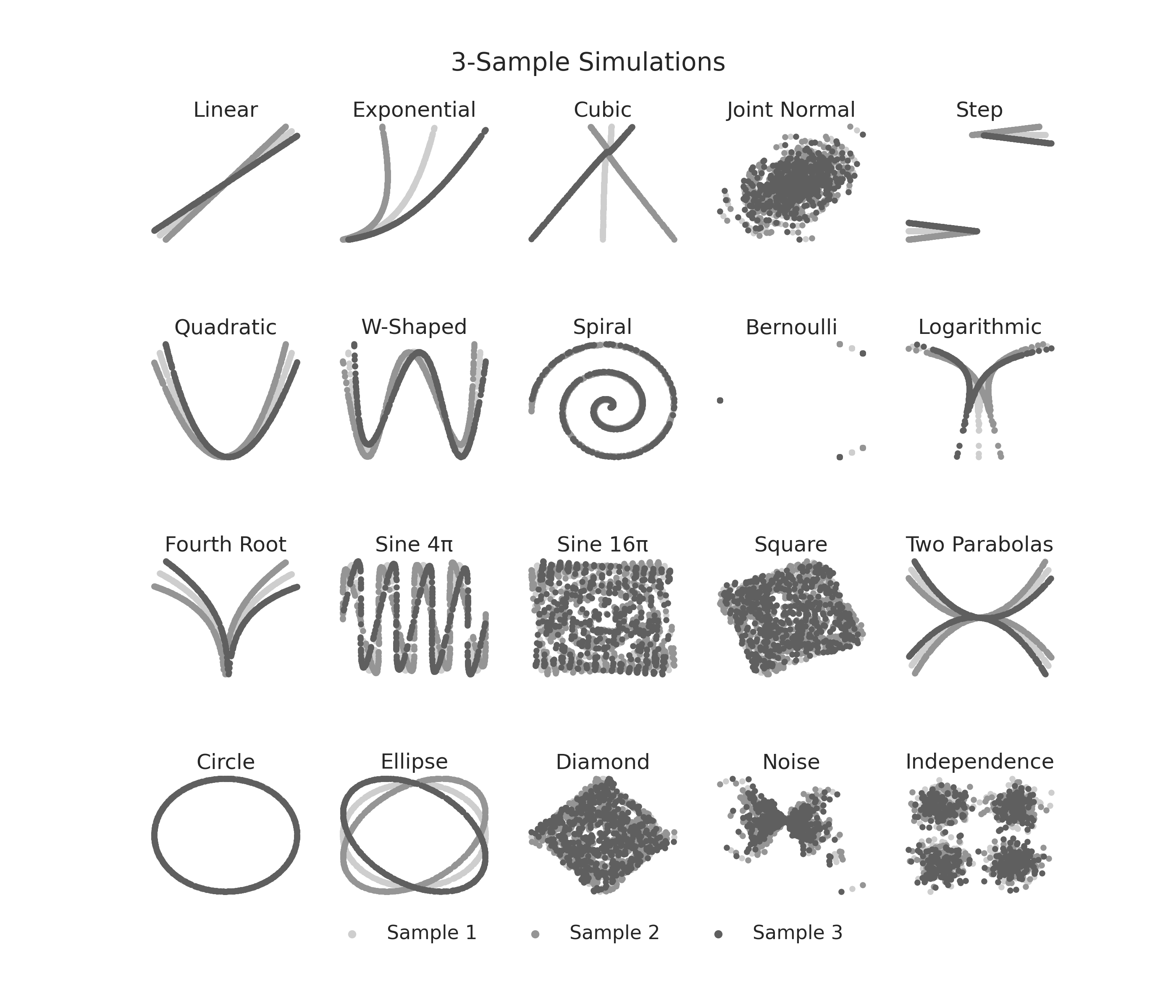# K-Sample Sims¶

K-sample simulations are found in `hyppo.tools`. Here, we visualize what these simulations look like. The original simulation and rotated simulation are shown. Note that since these are noise-free, we do not see any rotation due to the rotational symmetry of the simulation.

••```import matplotlib.pyplot as plt
import seaborn as sns
from hyppo.tools import SIMULATIONS, rot_ksamp

# make plots look pretty
sns.set(color_codes=True, style="white", context="talk", font_scale=2)
PALETTE = sns.color_palette("Greys", n_colors=9)
sns.set_palette(PALETTE[2::2])

# constants
N = 500  # sample size
P = 1  # dimensionality
DEGREE = [5, -5]  # angle

# simulation titles
SIM_TITLES = [
"Linear",
"Exponential",
"Cubic",
"Joint Normal",
"Step",
"W-Shaped",
"Spiral",
"Bernoulli",
"Logarithmic",
"Fourth Root",
"Sine 4\u03C0",
"Sine 16\u03C0",
"Square",
"Two Parabolas",
"Circle",
"Ellipse",
"Diamond",
"Noise",
"Independence",
]

# make a function that runs the code depending on the simulation
def plot_sims(k=2, degree=DEGREE):
"""Plot simulations"""
fig, ax = plt.subplots(nrows=4, ncols=5, figsize=(28, 24))

plt.suptitle("{}-Sample Simulations".format(k), y=0.93, va="baseline")

count = 0
for i, row in enumerate(ax):
for j, col in enumerate(row):
count = 5 * i + j
sim_title = SIM_TITLES[count]
sim = list(SIMULATIONS.keys())[count]

# rotated k-sample simulation
sims = rot_ksamp(sim, N, P, k=k, degree=degree, noise=False)

# plot the nose and noise-free sims
for index in range(len(sims)):
col.scatter(
sims[index][:, 0],
sims[index][:, 1],
label="Sample {}".format(index + 1),
)

# make the plot look pretty
col.set_title("{}".format(sim_title))
col.set_xticks([])
col.set_yticks([])
sns.despine(left=True, bottom=True, right=True)

leg = plt.legend(
bbox_to_anchor=(0.5, 0.1),
bbox_transform=plt.gcf().transFigure,
ncol=5,
loc="upper center",
)
leg.get_frame().set_linewidth(0.0)
for legobj in leg.legendHandles:
legobj.set_linewidth(5.0)